Function Repository Resource:

# SymmetricSort

Symmetrically reorder the rows and columns of a square matrix

Contributed by: Edmund B Robinson
 ResourceFunction["SymmetricSort"][matrix,from,to] symmetrically reorders the elements of matrix from ordering from to ordering to. ResourceFunction["SymmetricSort"][matrix,index] symmetrically reorders the elements of matrix by the ordering represented by index.

## Details

ResourceFunction["SymmetricSort"] maintains matrix symmetry when permuting the matrix elements.
ResourceFunction["SymmetricSort"] takes any expression that satisfies SquareMatrixQ for matrix.
In ResourceFunction["SymmetricSort"][matrix,from,to], from and to can be any expression that satisfies VectorQ and DuplicateFreeQ, and together satisfy ContainsOnly. matrix, from and to must all have the same length.
In ResourceFunction["SymmetricSort"][matrix,index], index can be any ordering represented as a permutation list or as a disjoint cycle.

## Examples

### Basic Examples (5)

Generate a covariance matrix from example data:

 In:=Out=The covariance matrix is symmetric and positive semidefinite:

 In:=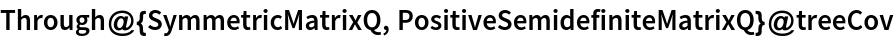Out=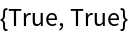A SymmetricSort using the variable names to change the order of the variables:

 In:=Out=Symmetric and positive semidefinite properties are preserved:

 In:=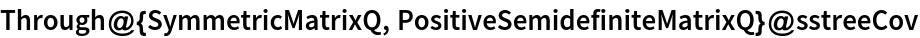Out=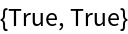SymmetricSort can also take an ordering of position indices:

 In:=Out=### Scope (2)

SymmetricSort preserves the diagonal and the relative positions of the upper and lower off-diagonals:

 In:=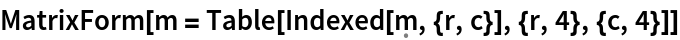Out=Diagonal elements in the original matrix remain on the diagonal in the reordered matrix, and off-diagonal relative positions are comparable. For example, m34 is still mirrored with m43:

 In:=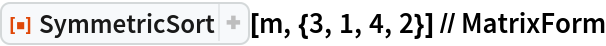Out=SymmetricSort accepts permutations expressed in cycle format:

 In:=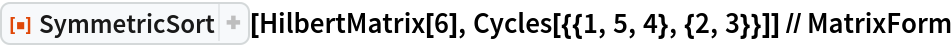Out=### Applications (2)

Properties of Graph, like WeightedAdjacencyMatrix, are given in the order of VertexList:

 In:=Out=SymmetricSort property elements in canonical order:

 In:=Out=In:=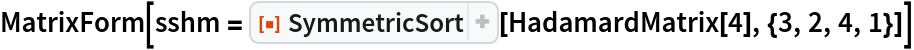Out=The permuted matrix is involutory, just like the original Hadamard matrix:

 In:=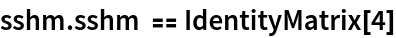Out=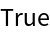### Properties and Relations (1)

The eigenvalues of a symmetric matrix are invariant under SymmetricSort:

 In:=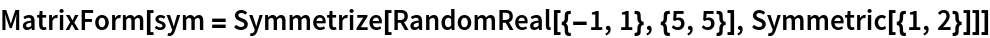Out=In:=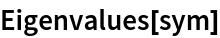Out=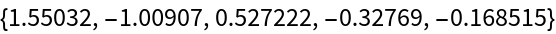In:=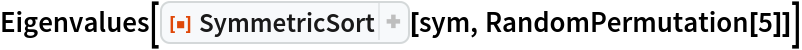Out=### Neat Examples (5)

An upper bidiagonal matrix:

 In:=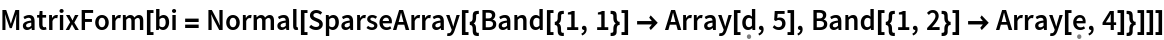Out=Generate a symmetric block matrix with bidiagonal blocks:

 In:=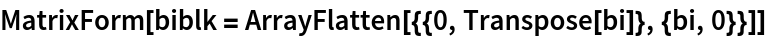Out=Use the resource function OutShuffle with SymmetricSort to transform the matrix into a tridiagonal matrix:

 In:=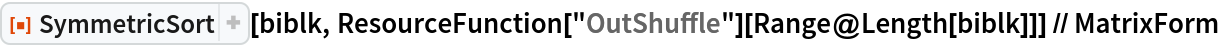Out=Compute the singular values of a numerical upper bidiagonal matrix:

 In:=Out=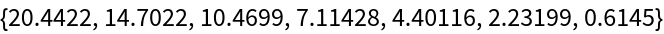These are equivalent to the positive eigenvalues of the permuted block matrix:

 In:=Out=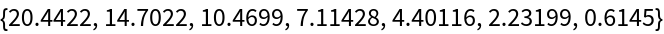## Publisher

Edmund B Robinson

## Version History

• 1.0.0 – 01 September 2021